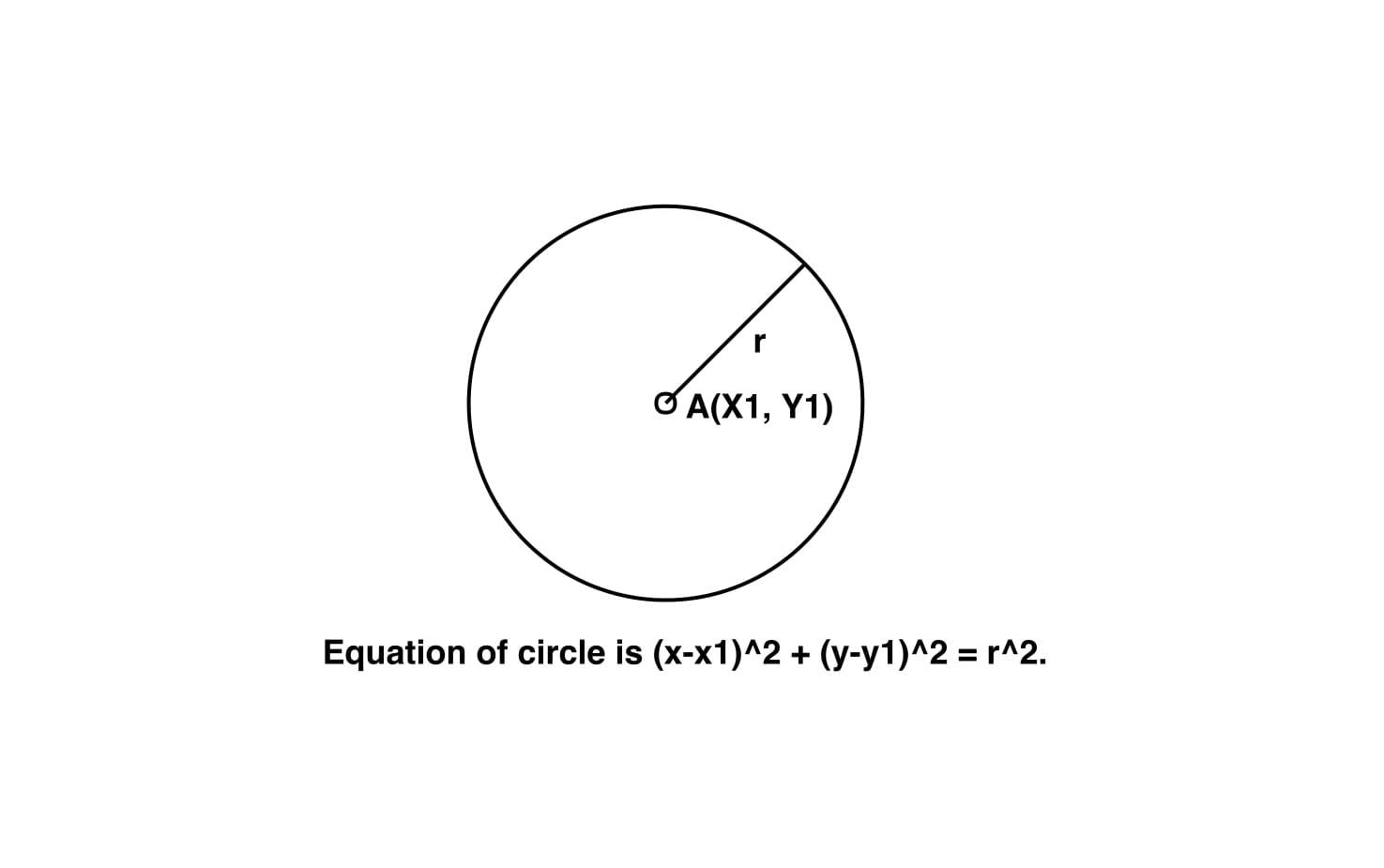GeeksforGeeks App
Open AppBrowser
Continue

# Equation of circle from center and radius

Given the center of circle (x1, y1) and its radius r, find the equation of the circle having center (x1, y1) and having radius r.
Examples:

Input : x1 = 2, y1 = -3, r = 8
Output : x^2 + y^2 – 4*x + 6*y = 51.
Input : x1 = 0, y1 = 0, r = 2
Output : x^2 + y^2 – 0*x + 0*y = 4.Approach:
Given the center of circle (x1, y1) and its radius r, we have to find the equation of the circle having center (x1, y1) and having radius r.
the equation of circle having center (x1, y1) and having radius r is given by :-

``

on expanding above equation

``

on arranging above we get

``

Below is the implementation of above approach:

## C++

 `// CPP program to find the equation``// of circle.``#include ``using` `namespace` `std;` `// Function to find the equation of circle``void` `circle_equation(``double` `x1, ``double` `y1, ``double` `r)``{``    ``double` `a = -2 * x1;` `    ``double` `b = -2 * y1;` `    ``double` `c = (r * r) - (x1 * x1) - (y1 * y1);` `    ``// Printing result``    ``cout << ``"x^2 + ("` `<< a << ``" x) + "``;``    ``cout << ``"y^2 + ("` `<< b << ``" y) = "``;``    ``cout << c << ``"."` `<< endl;``}` `// Driver code``int` `main()``{``    ``double` `x1 = 2, y1 = -3, r = 8;``    ``circle_equation(x1, y1, r);``    ``return` `0;``}`

## Java

 `// Java program to find the equation``// of circle.``import` `java.util.*;` `class` `solution``{` ` ``// Function to find the equation of circle``static` `void` `circle_equation(``double` `x1, ``double` `y1, ``double` `r)``{``    ``double` `a = -``2` `* x1;` `    ``double` `b = -``2` `* y1;` `    ``double` `c = (r * r) - (x1 * x1) - (y1 * y1);` `    ``// Printing result``    ``System.out.print(``"x^2 + ("` `+a+ ``" x) + "``);``     ``System.out.print(``"y^2 + ("``+b + ``" y) = "``);``     ``System.out.println(c +``"."` `);``}` `// Driver code``public` `static` `void` `main(String arr[])``{``    ``double` `x1 = ``2``, y1 = -``3``, r = ``8``;``    ``circle_equation(x1, y1, r);`` ` `}` `}`

## Python3

 `# Python3 program to find the``# equation of circle.` `# Function to find the``# equation of circle``def` `circle_equation(x1, y1, r):``    ``a ``=` `-``2` `*` `x1;` `    ``b ``=` `-``2` `*` `y1;` `    ``c ``=` `(r ``*` `r) ``-` `(x1 ``*` `x1) ``-` `(y1 ``*` `y1);` `    ``# Printing result``    ``print``(``"x^2 + ("``, a, ``"x) + "``, end ``=` `"");``    ``print``(``"y^2 + ("``, b, ``"y) = "``, end ``=` `"");``    ``print``(c, ``"."``);` `# Driver code``x1 ``=` `2``;``y1 ``=` `-``3``;``r ``=` `8``;``circle_equation(x1, y1, r);` `# This code is contributed``# by mits`

## C#

 `// C# program to find the equation``// of circle.``using` `System;` `class` `GFG``{` `// Function to find the equation of circle``public` `static` `void` `circle_equation(``double` `x1,``                                   ``double` `y1,``                                   ``double` `r)``{``    ``double` `a = -2 * x1;` `    ``double` `b = -2 * y1;` `    ``double` `c = (r * r) - (x1 * x1) - (y1 * y1);` `    ``// Printing result``    ``Console.Write(``"x^2 + ("` `+ a + ``" x) + "``);``    ``Console.Write(``"y^2 + ("``+ b + ``" y) = "``);``    ``Console.WriteLine(c + ``"."` `);``}` `// Driver code``public` `static` `void` `Main(``string` `[]arr)``{``    ``double` `x1 = 2, y1 = -3, r = 8;``    ``circle_equation(x1, y1, r);``}``}` `// This code is contributed``// by SoumkMondal`

## PHP

 ``

## Javascript

 ``

Output:

`x^2 + (-4 x) + y^2 + (6 y) = 51.`

Time complexity: O(1), since there is no loop or recursion.
Auxiliary Space: O(1), since no extra space has been taken.

My Personal Notes arrow_drop_up